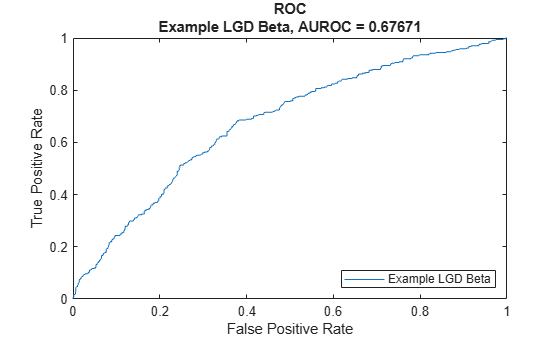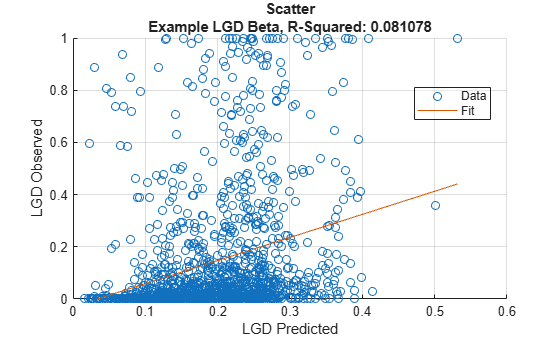# Beta

Create `Beta` model object for loss given default

Since R2022b

## Description

Create and analyze a `Beta` model object to calculate loss given default (LGD) using this workflow:

1. Use `fitLGDModel` to create a `Beta` model object.

2. Use `predict` to predict the LGD.

3. Use `modelDiscrimination` to return AUROC and ROC data. You can plot the results using `modelDiscriminationPlot`.

4. Use `modelCalibration` to return the R-squared, RMSE, correlation, and sample mean error of predicted and observed LGD data. You can plot the results using `modelCalibrationPlot`.

## Creation

### Syntax

``BetaLGDModel = fitLGDModel(data,ModelType)``
``BetaLGDModel = fitLGDModel(___,Name=Value)``

### Description

example

````BetaLGDModel = fitLGDModel(data,ModelType)` creates a `Beta` LGD model object.```

example

````BetaLGDModel = fitLGDModel(___,Name=Value)` specifies options using one or more name-value pair arguments in addition to the input arguments in the previous syntax. The optional name-value pair arguments set the model object properties. For example, ```BetaLGDModel = fitLGDModel(data,'Beta',PredictorVars={'LTV' 'Age' 'Type'},ResponseVar='LGD',BoundaryTolerance=1e-4)``` creates a `BetaLGDModel` object using a `Beta` model type. ```

### Input Arguments

expand all

Data for loss given default, specified as a table.

Data Types: `table`

Model type, specified as a string with the value of `"Beta"` or a character vector with the value of `'Beta'`.

Data Types: `char` | `string`

Name-Value Arguments

Specify optional pairs of arguments as `Name1=Value1,...,NameN=ValueN`, where `Name` is the argument name and `Value` is the corresponding value. Name-value arguments must appear after other arguments, but the order of the pairs does not matter.

Example: ```BetaLGDModel = fitLGDModel(data,'Beta',PredictorVars={'LTV' 'Age' 'Type'},ResponseVar='LGD',BoundaryTolerance=1e-4)```

User-defined model ID, specified as `ModelID` and a string or character vector. The software uses the `ModelID` text to format outputs and is expected to be short.

Data Types: `string` | `char`

User-defined description for model, specified as `Description` and a string or character vector.

Data Types: `string` | `char`

Predictor variables, specified as `PredictorVars` and a string array or cell array of character vectors. `PredictorVars` indicates which columns in the `data` input contain the predictor information. By default, `PredictorVars` is set to all the columns in the `data` input except for `ResponseVar`.

Data Types: `string` | `cell`

Response variable, specified as `ResponseVar` and a string or character vector. The response variable contains the LGD data and must be a numeric variable. An LGD value of `0` indicates no loss (full recovery), `1` indicates total loss (no recovery), and values between `0` and `1` indicate a partial loss. By default, `ResponseVar` is set to the last column.

Data Types: `string` | `char`

Value to perturb LGD response values away from 0 to 1, specified as `BoundaryTolerance` and a positive scalar numeric.

Data Types: `double`

Options for fitting, specified as `SolverOptions` and an `optimoptions` object that is created using `optimoptions` from Optimization Toolbox™. The defaults for the `optimoptions` object are:

• `"Display"``"none"`

• `"Algorithm"``"quasi-newton"`

• `"MaxFunctionEvaluations"``500` ✕ Number of model coefficients

• `"MaxIterations"` — 1000

Note

When using `optimoptions` with a `Beta` model, specify the `SolverName` as `fminunc`.

The number of `Beta` model coefficients is determined at run time, depending on the number of predictors and the number of categories in the categorical predictors.

Data Types: `object`

## Properties

expand all

User-defined model ID, returned as a string.

Data Types: `string`

User-defined description, returned as a string.

Data Types: `string`

Underlying statistical model, returned as a compact linear model object. The compact version of the underlying regression model is an instance of the `risk.internal.credit.BetaModel` class.

Data Types: `object`

Predictor variables, returned as a string array.

Data Types: `string`

Response variable, returned as a string.

Data Types: `string`

Value to perturb LGD response values away from 0 to 1, returned as a positive scalar numeric.

Data Types: `double`

## Object Functions

 `predict` Predict loss given default `modelDiscrimination` Compute AUROC and ROC data `modelDiscriminationPlot` Plot ROC curve `modelCalibration` Compute R-square, RMSE, correlation, and sample mean error of predicted and observed LGDs `modelCalibrationPlot` Scatter plot of predicted and observed LGDs

## Examples

collapse all

This example shows how to use `fitLGDModel` to create a `Beta` model object for loss given default (LGD).

```load LGDData.mat head(data)```
``` LTV Age Type LGD _______ _______ ___________ _________ 0.89101 0.39716 residential 0.032659 0.70176 2.0939 residential 0.43564 0.72078 2.7948 residential 0.0064766 0.37013 1.237 residential 0.007947 0.36492 2.5818 residential 0 0.796 1.5957 residential 0.14572 0.60203 1.1599 residential 0.025688 0.92005 0.50253 investment 0.063182 ```
```rng('default'); NumObs = height(data); c = cvpartition(NumObs,'HoldOut',0.4); TrainingInd = training(c); TestInd = test(c);```

Create `Beta` LGD Model

Use `fitLGDModel` to create a `Beta` model object using the `TrainingInd` data.

```BetaLGDModel = fitLGDModel(data(TrainingInd,:),'Beta',... ModelID='Example LGD Beta',... PredictorVars={'LTV' 'Age' 'Type'},... ResponseVar='LGD',... BoundaryTolerance=2e-05); disp(BetaLGDModel)```
``` Beta with properties: BoundaryTolerance: 2.0000e-05 ModelID: "Example LGD Beta" Description: "" UnderlyingModel: [1x1 risk.internal.credit.BetaModel] PredictorVars: ["LTV" "Age" "Type"] ResponseVar: "LGD" ```

Display the underlying model.

`disp(BetaLGDModel.UnderlyingModel)`
```Beta regression model: logit(LGD) ~ 1_mu + LTV_mu + Age_mu + Type_mu log(LGD) ~ 1_phi + LTV_phi + Age_phi + Type_phi Estimated coefficients: Estimate SE tStat pValue ________ ________ ________ __________ (Intercept)_mu -1.3884 0.13116 -10.585 0 LTV_mu 0.61404 0.14989 4.0967 4.3502e-05 Age_mu -0.47446 0.039808 -11.919 0 Type_investment_mu 0.4516 0.084719 5.3305 1.0846e-07 (Intercept)_phi -0.11299 0.12578 -0.89834 0.36911 LTV_phi 0.030895 0.14692 0.21028 0.83347 Age_phi 0.23972 0.040152 5.9702 2.7758e-09 Type_investment_phi -0.14724 0.078187 -1.8831 0.059821 Number of observations: 2093 Log-likelihood: -4976.51 ```

Predict LGD

For `Beta` models, use `predict` to calculate the predicted LGD value, which is the unconditional expected value of the response, given the predictor values.

`predictedLGD = predict(BetaLGDModel,data(TestInd,:))`
```predictedLGD = 1394×1 0.0935 0.1483 0.3520 0.0963 0.1879 0.2587 0.2671 0.0212 0.1768 0.2249 ⋮ ```

Validate LGD Model

Use `modelDiscriminationPlot` to plot the ROC curve.

`modelDiscriminationPlot(BetaLGDModel,data(TestInd,:))`Use `modelCalibrationPlot` to show a scatter plot of the predictions.

`modelCalibrationPlot(BetaLGDModel,data(TestInd,:))`